# Learning Electronics

Learn to build electronic circuits

# Digital codes

### Question 1:

Counting practice: count from zero to thirty-one in binary, octal, and hexadecimal: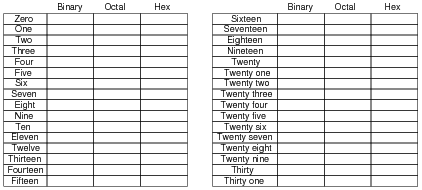No answers given here - compare with your classmates!

Notes:
In order to familiarize students with these ßtrange" numeration systems, I like to begin each day of digital circuit instruction with counting practice. Students need to be fluent in these numeration systems by the time they are finished studying digital circuits!
One suggestion I give to students to help them see patterns in the count sequences is "pad" the numbers with leading zeroes so that all numbers have the same number of characters. For example, instead of writing "10" for the binary number two, write "00010". This way, the patterns of character cycling (especially binary, where each successively higher-valued bit has half the frequency of the one before it) become more evident to see.

### Question 2:

Rotary encoders are electromechanical devices used to convert an angular position (shaft rotation) into a digital signal. The simplest form of rotary encoder uses a slotted wheel with a single LED/photodetector pair to generate pulses as the wheel turns: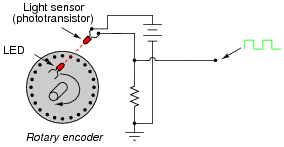Some rotary encoder designs have multiple-bit outputs, with each LED/photodetector pair reading a different "track" of slots in the disk: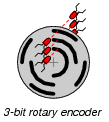In the illustration shown above, identify which LED/photodetector pairs represent the MSB (Most Significant Bit) and LSB (Least Significant Bit) of the binary output. Also, identify which direction the wheel must turn in order to produce an increasing count.
Note: assume that the darkest areas on the illustration represent slots cut through the disk, while the grey areas represent parts of the disk that are opaque.
I'll let you figure out the MSB, LSB, and up-count direction on your own! It isn't difficult to do if you have mastered counting in binary.

Notes:
Ask your students to brainstorm possible applications for rotary encoders. Where might we use such a device? Also, ask them to contrast the two encoder types (1 bit versus 3-bit) shown in the question. What applications might demand the 3-bit, versus only require a 1-bit encoder?

### Question 3:

Absolute rotary encoders often use a code known as Gray code rather than binary, to represent angular position. This code was patented by Frank Gray of Bell Labs in 1953, as a means of reducing errors in rotary encoder output. Examine each of these encoder disks, and determine which one is binary and which one is Gray code: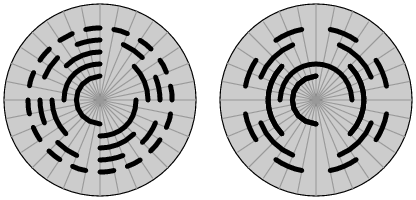Assuming that the darkest areas on the illustration represent slots cut through the disk, and the grey areas represent parts of the disk that are opaque, mark the "zero," öne," and "two" sectors on each disk.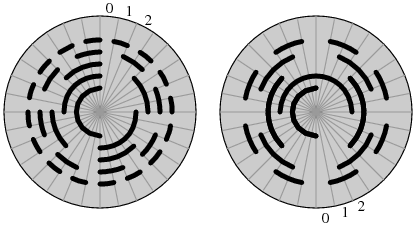I won't directly tell you which disk is which, but I will provide a comparison of 5-bit binary versus Gray code, to help you in your analysis:
 Binary Gray
 00000 00000
 00001 00001
 00010 00011
 00011 00010
 00100 00110
 00101 00111
 00110 00101
 00111 00100
 01000 01100
 01001 01101
 01010 01111
 01011 01110
 01100 01010
 01101 01011
 01110 01001
 01111 01000
 10000 11000
 10001 11001
 10010 11011
 10011 11010
 10100 11110
 10101 11111
 10110 11101
 10111 11100
 11000 10100
 11001 10101
 11010 10111
 11011 10110
 11100 10010
 11101 10011
 11110 10001
 11111 10000

Notes:
Ask your students what patterns they notice in the Gray code sequence, as compared to the binary count. What difference do they see between binary and Gray code, analyzing the bit transitions from one number to the next?

### Question 4:

Explain why Gray code is often used in rotary encoders rather than binary coding. What difference does it make what type of code we use to mark the sectors of an encoder disk, so long as each sector possesses a unique number?
Gray code markings are more tolerant of sensor misalignment than binary markings, because there is no need for perfect synchronization of multiple bit transitions between sectors.

Notes:
This is perhaps the most important reason for using Gray code in encoder marking, but it is not necessarily obvious why to the new student. I found that making a physical mock-up of a binary-coded wheel versus a Gray-coded wheel helped me better present this concept to students. Those students with better visualization/spatial relations skills will grasp this concept faster than the others, so you might want to solicit their help in explaining it to the rest of the class.

### Question 5:

Convert the following binary numbers into Gray code:

1001102 =
1100102 =
1010012 =
10101001102 =

1001102 = 110101Gray
1100102 = 101011Gray
1010012 = 111101Gray
10101001102 = 1111110101Gray

Notes:
There are many textbook references for the conversion process between binary and Gray code. Let your students research how the conversions are done!

### Question 6:

Convert the following Gray code numbers into binary:

111110Gray =
100001Gray =
101110Gray =
1110001111Gray =

111110Gray = 1010112
100001Gray = 1111102
101110Gray = 1101002
1110001111Gray = 10111101012

Notes:
There are many textbook references for the conversion process between binary and Gray code. Let your students research how the conversions are done!

### Question 7:

A nearly universal standard for representing text data in digital form is the ASCII code. What does the acronym ÄSCII" stand for, and what is the format of this code?
ÄSCII" = American Standard Code for Information Interchange. Basic ASCII is a seven-bit binary code capable of representing all alphabetical characters used in the English language (upper-case as well as lower), as well as Arabic numerals, English punctuation marks, and some miscellaneous control codes for teletype machines.
Challenge question: although ASCII technically requires only 7 bits, a full 8 bits (1 byte) is usually reserved for each ASCII character in computer systems. Explain why.

Notes:
ASCII is arguably the lingua franca of the digital world. Despite its humble beginnings and Anglo-centric format, it is used worldwide in digital computer and telecommunication systems. Let your students know that every plain-text computer file is nothing more than a collection of ASCII codes, one code for each text character (including spaces).

### Question 8:

Decode this set of ASCII characters, to reveal a secret message (all codes given in hexadecimal format):
49 20 4C 6F 76 65 20 45 6C 65 63 74 72 6F 6E 69 63 73 21
I'll let you decode this message on your own!

Notes:
This question provides students with practice using an ASCII reference table.

### Question 9:

Explain why Binary Coded Decimal (BCD) is sometimes referred to as the "8421" code. Why is this code used at all?
BCD uses groups of four binary bits to represent each digit of a decimal number. The LSD place weights are 8-4-2-1, while the next significant digit's place weightings are 80-40-20-10, and so on.
Follow-up question: the four bits used for each BCD character could be called half of a byte (8 bits). There is a special word for a four-bit grouping. What is that word?

Notes:
Discuss with your students the purpose of using BCD to represent decimal quantities. While not an efficient usage of bits, BCD certainly is convenient for representing decimal figures with discrete (0 or 1) logic states.

### Question 10:

Convert the following decimal numbers into BCD form:

73910
2510
9224110

Convert the following BCD numbers into decimal form:

1000 1001
0100 0111 0110
0011 1000 0101 0001

73910 = 0111 0011 1001
2510 = 0010 0101
9224110 = 1001 0010 0010 0100 0001

1000 1001 = 8910
0100 0111 0110 = 47610
0011 1000 0101 0001 = 385110

Notes:
Nothing but straightforward conversions here!

### Question 11:

Explain how the Morse Code resembles ASCII in structure and purpose.
Morse Code is digital, being composed of only two types of characters, just like ASCII. Also, its purpose is to convey alphanumeric information, just like ASCII.

Notes:
An interesting point to bring up to students about Morse Code is that it is self-compressing. Note how different Morse characters possess different "bit" lengths, whereas ASCII characters are all 7 bits each (or 8 bits for Extended ASCII). This makes Morse a more efficient code than ASCII, from the perspective of bit economy!
Ask your students what ramifications this ßelf-compressing" aspect of Morse Code would have if we were to choose it over ASCII for sending alphanumeric characters over digital communications lines, or store alphanumeric characters in some form of digital memory media.

### Question 12:

Standard ASCII codes are seven bits in length, but communications equipment usually sends data in eight-bit (byte) groups. The extra bit is often used as a parity bit. What is the purpose of a "parity bit," and how is it used?
"Parity bits" are used as a primitive form of error detection. Communications equipment making use of parity for error detection may either be configured for ëven parity" or for ödd parity". I'll let you research and explain how parity bits are used in the transmission of ASCII data.

Notes:
The concept of parity is not very complex. It should be well within the reach of students to research on their own and report their findings to the class as a whole.